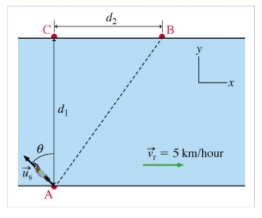# Problem: A swimmer wants to cross a river, from point A to point B, as shown in the figure. The distance d1 (from A to C) is 200 m, the distance d2 (from C to B) is 150 m, and the speed vr of the current in the river is 5 km/hour. Suppose that the swimmer's velocity relative to the water makes an angle of θ = 45 degrees with the line from A to C, as indicated in the figure.To swim directly from A to B, what speed us, relative to the water, should the swimmer have?Express the swimmer's speed numerically, to three significant figures, in kilometers per hour.

###### FREE Expert Solution

In this question, we'll determine the resultant x and y components of velocity.

We'll determine the time taken to cover the d1 and d2 in terms of the variables given. The times are equal, and hence we'll be able to determine the unknown us

The swimmer has both x and y components of velocity. Remember, θ is measured from the y-axis.

usy = vscos45

usx = -vssin45

vry = 0 m/s

vrx = 5 km/h

d1 = 200 m(1km/1000m) = 0.20 km

79% (457 ratings)###### Problem Details

A swimmer wants to cross a river, from point A to point B, as shown in the figure. The distance d1 (from A to C) is 200 m, the distance d2 (from C to B) is 150 m, and the speed vr of the current in the river is 5 km/hour. Suppose that the swimmer's velocity relative to the water makes an angle of θ = 45 degrees with the line from A to C, as indicated in the figure.To swim directly from A to B, what speed us, relative to the water, should the swimmer have?

Express the swimmer's speed numerically, to three significant figures, in kilometers per hour.